# Map Reduce Map Reduce Outline Map Reduce Architecture

• Slides: 43Map. ReduceMap. Reduce Outline • • Map. Reduce Architecture Map. Reduce Internals Map. Reduce Examples Job. Tracker Interface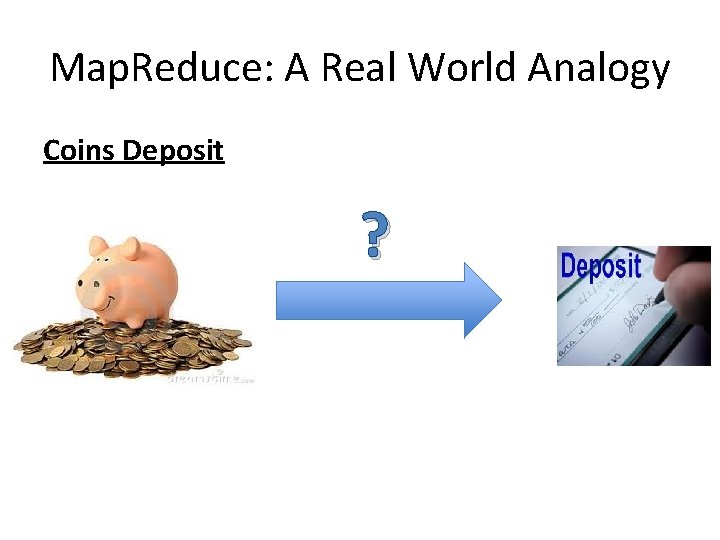Map. Reduce: A Real World Analogy Coins Deposit ?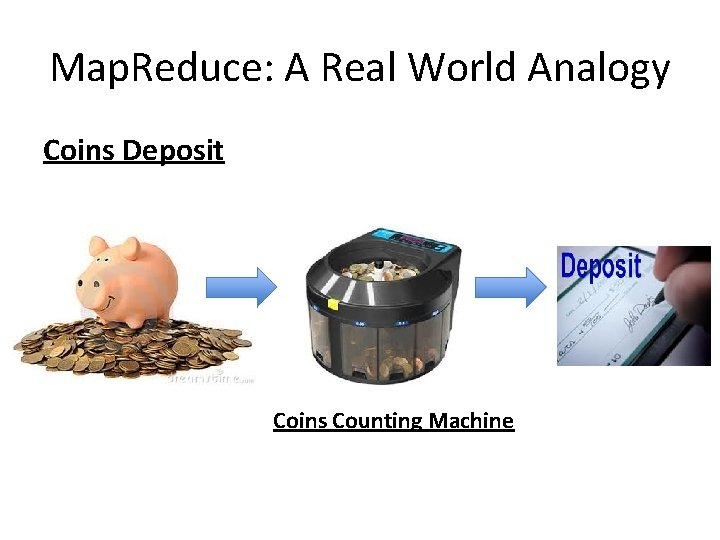Map. Reduce: A Real World Analogy Coins Deposit Coins Counting MachineMap. Reduce: A Real World Analogy Coins Deposit Mapper: Categorize coins by their face values Reducer: Count the coins in each face value in parallelMap. Reduce Architecture: Master-Slaves Job Client Task Trackers Job Tracker Idea: Bring Computation to Data! Map Reduce Name Node Job Client: Submit Jobs Inputs HDFS Output s Task Tracker: Execute Jobs Job Tracker: Coordinate Jobs Job: Map. Reduce Function+ Config (Scheduling, Phase Coordination, etc. )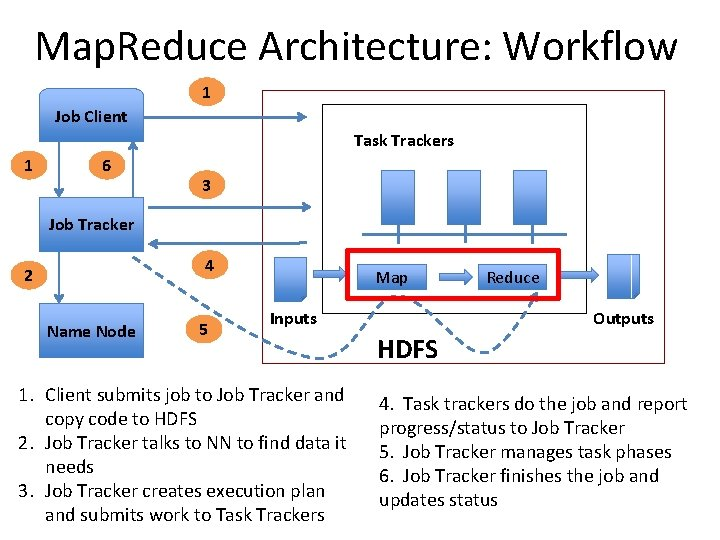Map. Reduce Architecture: Workflow 1 Job Client Task Trackers 1 6 3 Job Tracker 4 2 Name Node 5 Map Inputs 1. Client submits job to Job Tracker and copy code to HDFS 2. Job Tracker talks to NN to find data it needs 3. Job Tracker creates execution plan and submits work to Task Trackers Reduce Outputs HDFS 4. Task trackers do the job and report progress/status to Job Tracker 5. Job Tracker manages task phases 6. Job Tracker finishes the job and updates statusMap. Reduce Paradigm • Implement two functions: – Map (k 1, v 1) -> list (k 2, v 2) – Reduce(k 2, list(v 2)) -> list (v 3) • Framework handles everything else • Value with the same key go to the same reducer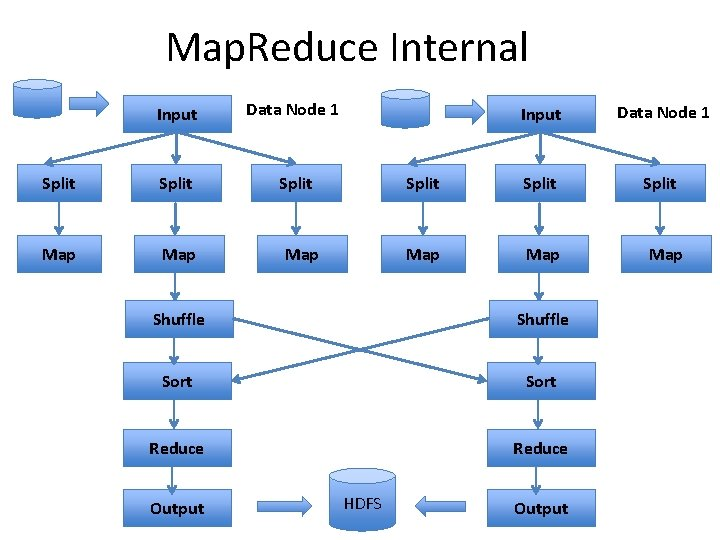Map. Reduce Internal Input Data Node 1 Split Map Map Input Data Node 1 Split Map Shuffle Sort Reduce Output HDFS Output MapMap. Reduce Example: Word Count Input Deer Beer River Car River Deer Car Beer Split Map Shuttle/Sort Reduce Dear Beer River Deer, 1 Beer, 1 River, 1 Beer, 2 Car River Car, 1 River, 1 Car, 3 Deer Car Beer Deer, 1 Car, 1 Beer, 1 Deer, 2 River, 1 River, 2 Similar Flavor of Coins Deposit ? Output Beer, 2 Car, 3 Deer, 2 River, 2Map. Reduce Example: Word Count Input Deer Beer River Car River Deer Car Beer Split Map Shuttle/Sort Reduce Dear Beer River Deer, 1 Beer, 1 River, 1 Beer, 2 Car River Car, 1 River, 1 Car, 3 Deer Car Beer Deer, 1 Car, 1 Beer, 1 Deer, 2 River, 1 River, 2 Q: What are the Key and Value Pairs of Map and Reduce? Map: Key=word, Value=1 Reduce: Key=word, Value=aggregated count Output Beer, 2 Car, 3 Deer, 2 River, 2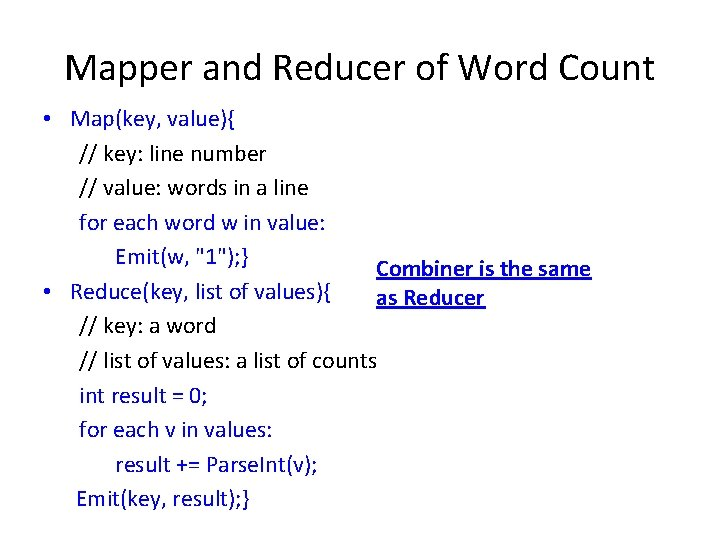Mapper and Reducer of Word Count • Map(key, value){ // key: line number // value: words in a line for each word w in value: Emit(w, "1"); } Combiner is the same • Reduce(key, list of values){ as Reducer // key: a word // list of values: a list of counts int result = 0; for each v in values: result += Parse. Int(v); Emit(key, result); }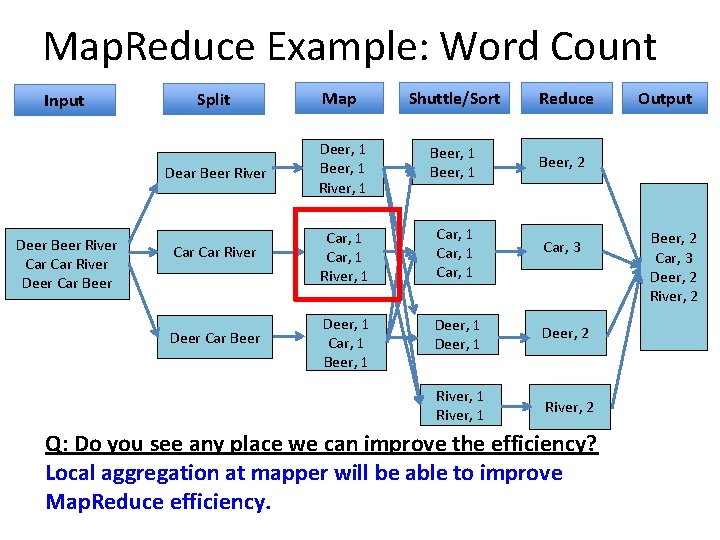Map. Reduce Example: Word Count Input Deer Beer River Car River Deer Car Beer Split Map Shuttle/Sort Reduce Dear Beer River Deer, 1 Beer, 1 River, 1 Beer, 2 Car River Car, 1 River, 1 Car, 3 Deer Car Beer Deer, 1 Car, 1 Beer, 1 Deer, 2 River, 1 River, 2 Q: Do you see any place we can improve the efficiency? Local aggregation at mapper will be able to improve Map. Reduce efficiency. Output Beer, 2 Car, 3 Deer, 2 River, 2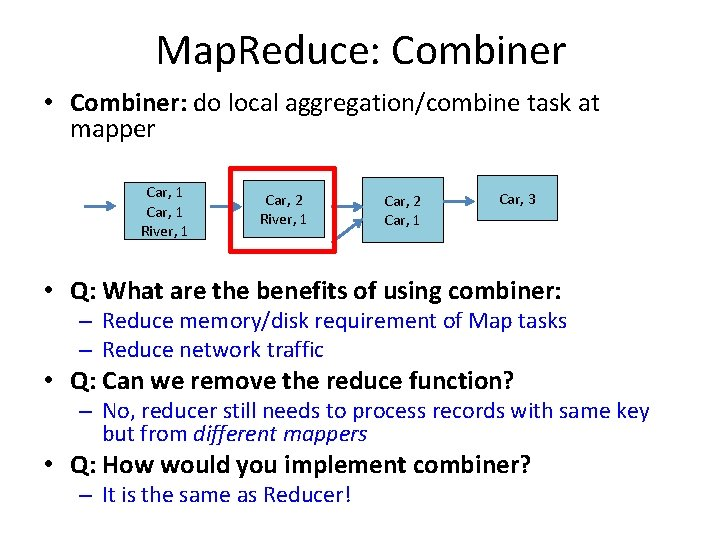Map. Reduce: Combiner • Combiner: do local aggregation/combine task at mapper Car, 1 River, 1 Car, 2 Car, 1 Car, 3 • Q: What are the benefits of using combiner: – Reduce memory/disk requirement of Map tasks – Reduce network traffic • Q: Can we remove the reduce function? – No, reducer still needs to process records with same key but from different mappers • Q: How would you implement combiner? – It is the same as Reducer!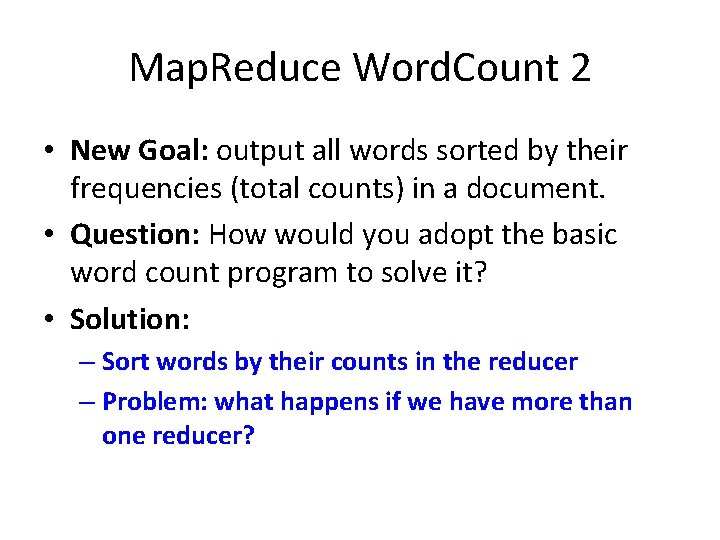Map. Reduce Word. Count 2 • New Goal: output all words sorted by their frequencies (total counts) in a document. • Question: How would you adopt the basic word count program to solve it? • Solution: – Sort words by their counts in the reducer – Problem: what happens if we have more than one reducer?Map. Reduce Word. Count 2 • New Goal: output all words sorted by their frequencies (total counts) in a document. • Question: How would you adopt the basic word count program to solve it? • Solution: – Do two rounds of Map. Reduce – In the 2 nd round, take the output of Word. Count as input but switch key and value pair! – Leverage the sorting capability of shuffle/sort to do the global sorting!Map. Reduce Word. Count 3 • New Goal: output the top K words sorted by their frequencies (total counts) in a document. • Question: How would you adopt the basic word count program to solve it? • Solution: – Use the solution of previous problem and only grab the top K in the final output – Problem: is there a more efficient way to do it?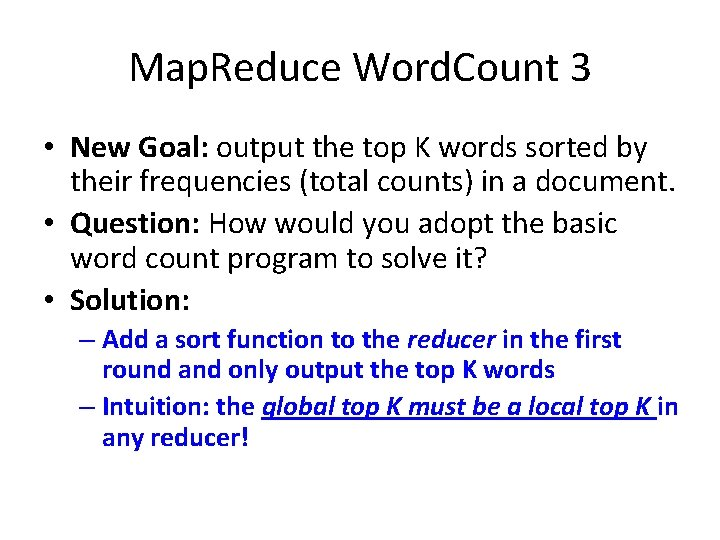Map. Reduce Word. Count 3 • New Goal: output the top K words sorted by their frequencies (total counts) in a document. • Question: How would you adopt the basic word count program to solve it? • Solution: – Add a sort function to the reducer in the first round and only output the top K words – Intuition: the global top K must be a local top K in any reducer!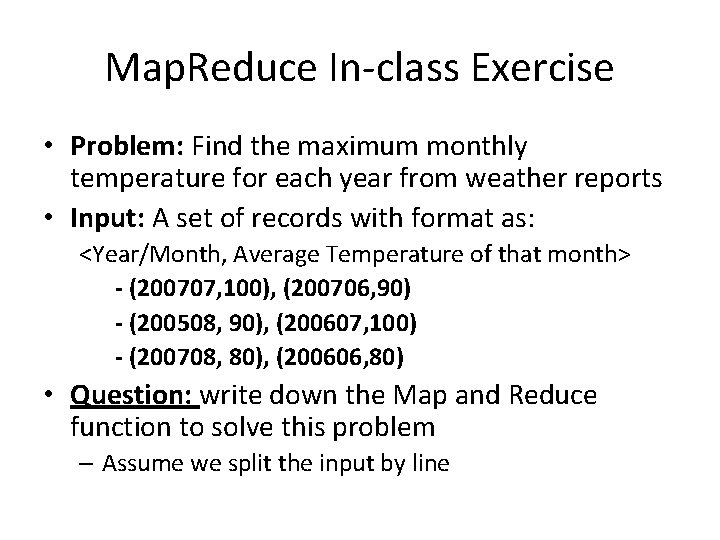Map. Reduce In-class Exercise • Problem: Find the maximum monthly temperature for each year from weather reports • Input: A set of records with format as: <Year/Month, Average Temperature of that month> - (200707, 100), (200706, 90) - (200508, 90), (200607, 100) - (200708, 80), (200606, 80) • Question: write down the Map and Reduce function to solve this problem – Assume we split the input by line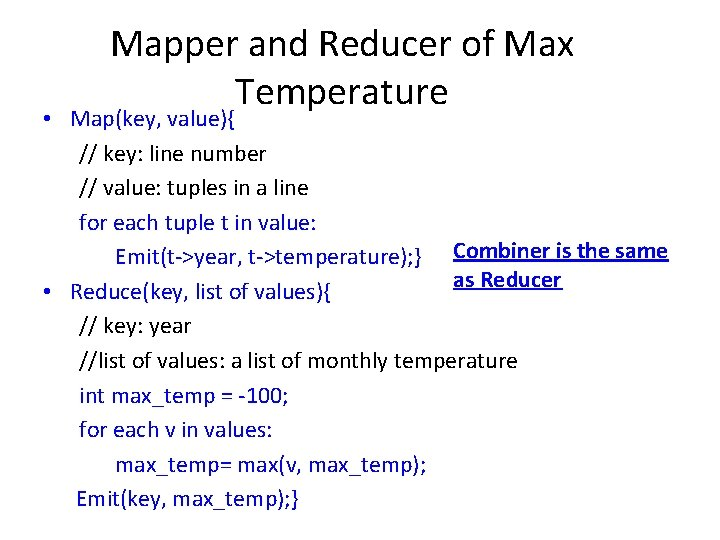Mapper and Reducer of Max Temperature • Map(key, value){ // key: line number // value: tuples in a line for each tuple t in value: Emit(t->year, t->temperature); } Combiner is the same as Reducer • Reduce(key, list of values){ // key: year //list of values: a list of monthly temperature int max_temp = -100; for each v in values: max_temp= max(v, max_temp); Emit(key, max_temp); }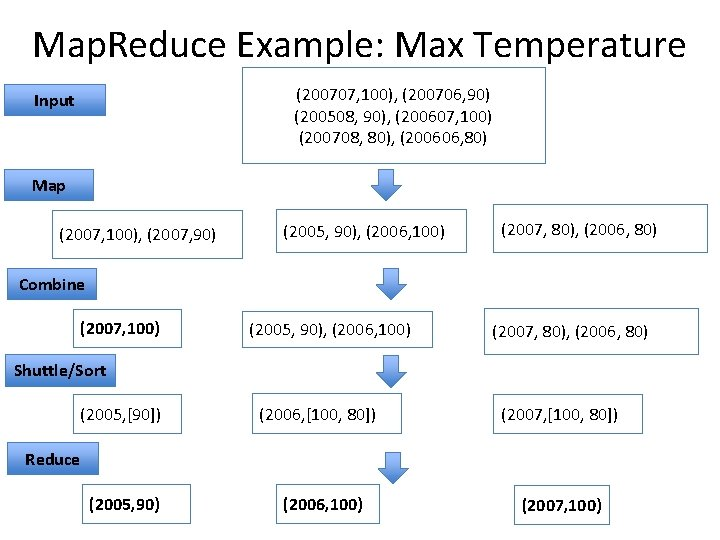Map. Reduce Example: Max Temperature (200707, 100), (200706, 90) (200508, 90), (200607, 100) (200708, 80), (200606, 80) Input Map (2007, 100), (2007, 90) (2005, 90), (2006, 100) (2007, 80), (2006, 80) Combine (2007, 100) (2005, 90), (2006, 100) (2007, 80), (2006, 80) Shuttle/Sort (2005, ) (2006, [100, 80]) (2007, [100, 80]) (2006, 100) (2007, 100) Reduce (2005, 90)Map. Reduce In-class Exercise • Key-Value Pair of Map and Reduce: – Map: (year, temperature) – Reduce: (year, maximum temperature of the year) • Question: How to use the above Map Reduce program (that contains the combiner) with slight changes to find the average monthly temperature of the year?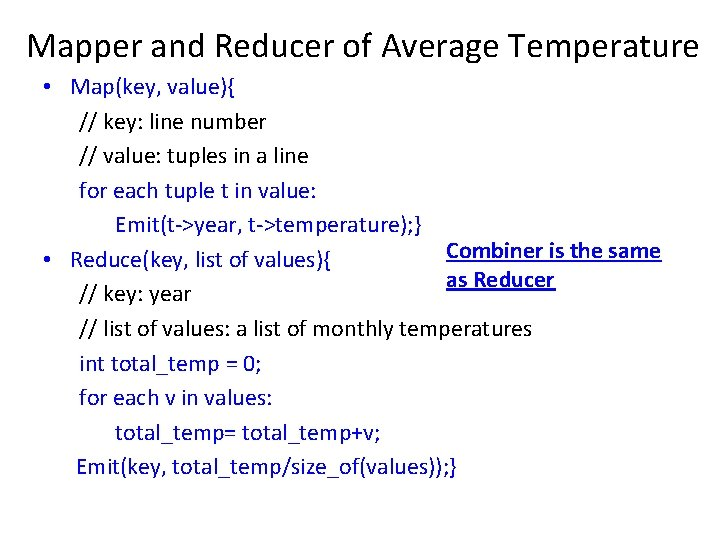Mapper and Reducer of Average Temperature • Map(key, value){ // key: line number // value: tuples in a line for each tuple t in value: Emit(t->year, t->temperature); } Combiner is the same • Reduce(key, list of values){ as Reducer // key: year // list of values: a list of monthly temperatures int total_temp = 0; for each v in values: total_temp= total_temp+v; Emit(key, total_temp/size_of(values)); }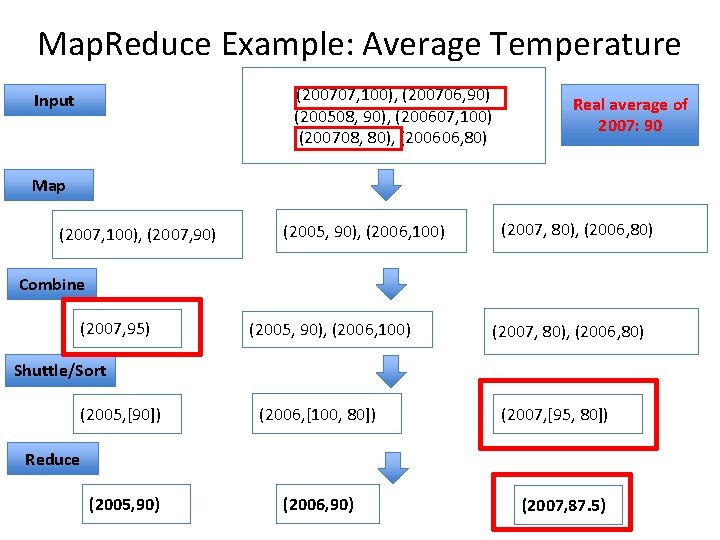Map. Reduce Example: Average Temperature (200707, 100), (200706, 90) (200508, 90), (200607, 100) (200708, 80), (200606, 80) Input Real average of 2007: 90 Map (2007, 100), (2007, 90) (2005, 90), (2006, 100) (2007, 80), (2006, 80) Combine (2007, 95) (2005, 90), (2006, 100) (2007, 80), (2006, 80) Shuttle/Sort (2005, ) (2006, [100, 80]) (2007, [95, 80]) Reduce (2005, 90) (2006, 90) (2007, 87. 5)Map. Reduce In-class Exercise • The problem is with the combiner! • Here is a simple counterexample: – (2007, 100), (2007, 90) -> (2007, 95) (2007, 80)->(2007, 80) – Average of the above is: (2007, 87. 5) – However, the real average is: (2007, 90) • However, we can do a small trick to get around this – Mapper: (2007, 100), (2007, 90) -> (2007, <190, 2>) (2007, 80)->(2007, <80, 1>) – Reducer: (2007, <270, 3>)->(2007, 90)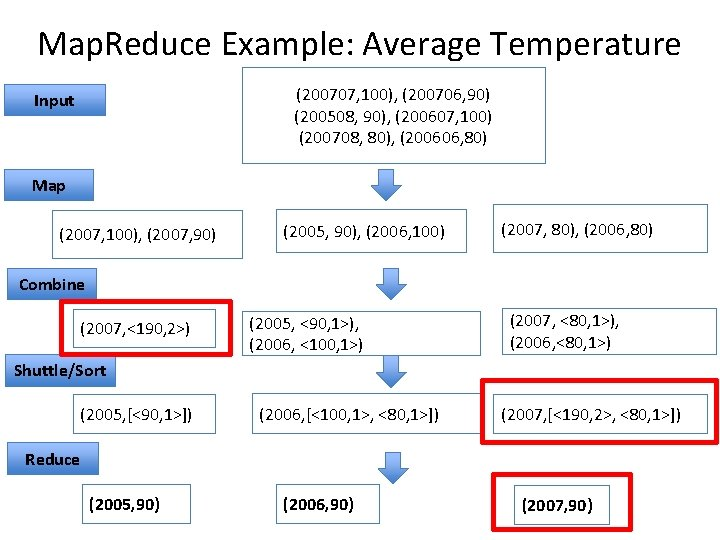Map. Reduce Example: Average Temperature (200707, 100), (200706, 90) (200508, 90), (200607, 100) (200708, 80), (200606, 80) Input Map (2007, 100), (2007, 90) (2005, 90), (2006, 100) (2007, 80), (2006, 80) Combine (2007, <190, 2>) (2005, <90, 1>), (2006, <100, 1>) (2007, <80, 1>), (2006, <80, 1>) Shuttle/Sort (2005, [<90, 1>]) (2006, [<100, 1>, <80, 1>]) (2007, [<190, 2>, <80, 1>]) Reduce (2005, 90) (2006, 90) (2007, 90)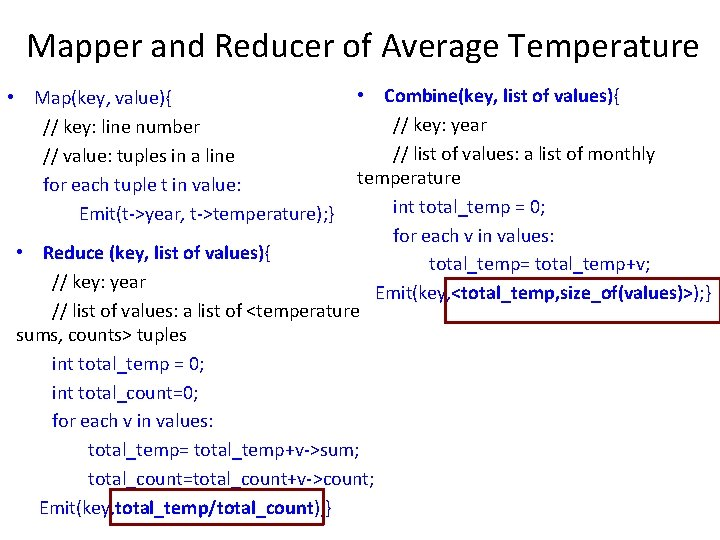Mapper and Reducer of Average Temperature • Combine(key, list of values){ // key: year // list of values: a list of monthly temperature int total_temp = 0; for each v in values: • Reduce (key, list of values){ total_temp= total_temp+v; // key: year Emit(key, <total_temp, size_of(values)>); } // list of values: a list of <temperature sums, counts> tuples int total_temp = 0; int total_count=0; for each v in values: total_temp= total_temp+v->sum; total_count=total_count+v->count; Emit(key, total_temp/total_count); } • Map(key, value){ // key: line number // value: tuples in a line for each tuple t in value: Emit(t->year, t->temperature); }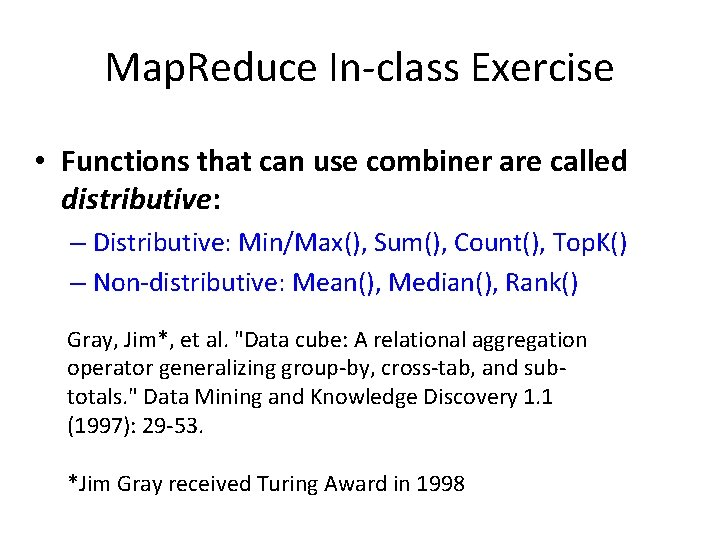Map. Reduce In-class Exercise • Functions that can use combiner are called distributive: – Distributive: Min/Max(), Sum(), Count(), Top. K() – Non-distributive: Mean(), Median(), Rank() Gray, Jim*, et al. "Data cube: A relational aggregation operator generalizing group-by, cross-tab, and subtotals. " Data Mining and Knowledge Discovery 1. 1 (1997): 29 -53. *Jim Gray received Turing Award in 1998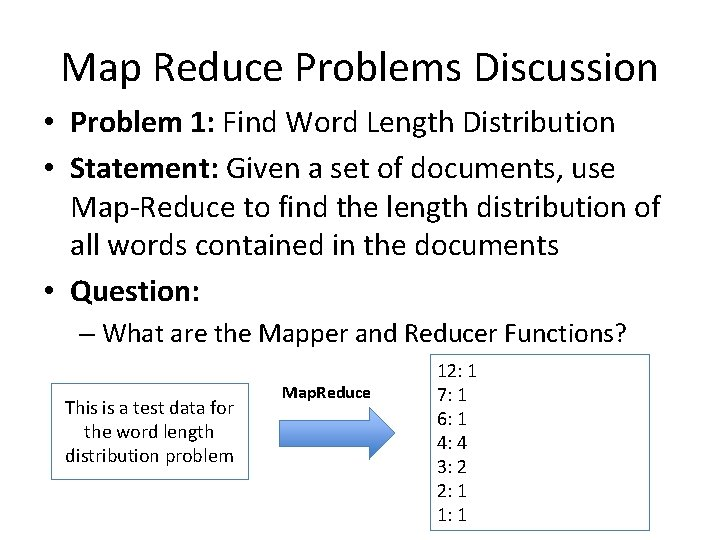Map Reduce Problems Discussion • Problem 1: Find Word Length Distribution • Statement: Given a set of documents, use Map-Reduce to find the length distribution of all words contained in the documents • Question: – What are the Mapper and Reducer Functions? This is a test data for the word length distribution problem Map. Reduce 12: 1 7: 1 6: 1 4: 4 3: 2 2: 1 1: 1Mapper and Reducer of Word Length Distribution • Map(key, value){ // key: document name // value: words in a document for each word w in value: Emit(length(w), w); } • Reduce(key, list of values){ // key: length of a word // list of values: a list of words with the same length Emit(key, size_of(values)); }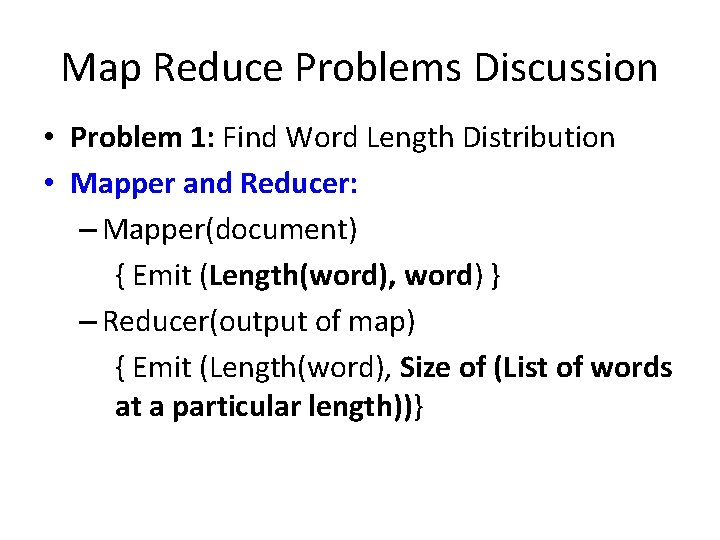Map Reduce Problems Discussion • Problem 1: Find Word Length Distribution • Mapper and Reducer: – Mapper(document) { Emit (Length(word), word) } – Reducer(output of map) { Emit (Length(word), Size of (List of words at a particular length))}Map Reduce Problems Discussion • Problem 2: Indexing & Page Rank • Statement: Given a set of web pages, each page has a page rank associated with it, use Map-Reduce to find, for each word, a list of pages (sorted by rank) that contains that word • Question: – What are the Mapper and Reducer Functions? Map. Reduce Word 1: [page x 1, page x 2, . . ] Word 2: [page y 1, page y 2, …] …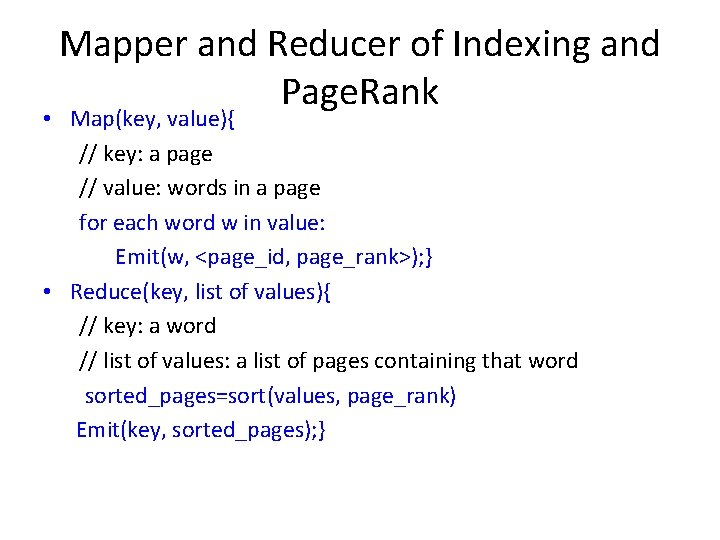Mapper and Reducer of Indexing and Page. Rank • Map(key, value){ // key: a page // value: words in a page for each word w in value: Emit(w, <page_id, page_rank>); } • Reduce(key, list of values){ // key: a word // list of values: a list of pages containing that word sorted_pages=sort(values, page_rank) Emit(key, sorted_pages); }Map Reduce Problems Discussion • Problem 2: Indexing and Page Rank • Mapper and Reducer: – Mapper(page_id, <page_text, page_rank>) { Emit (word, <page_id, page_rank>) } – Reducer(output of map) { Emit (word, List of pages contains the word sorted by their page_ranks)}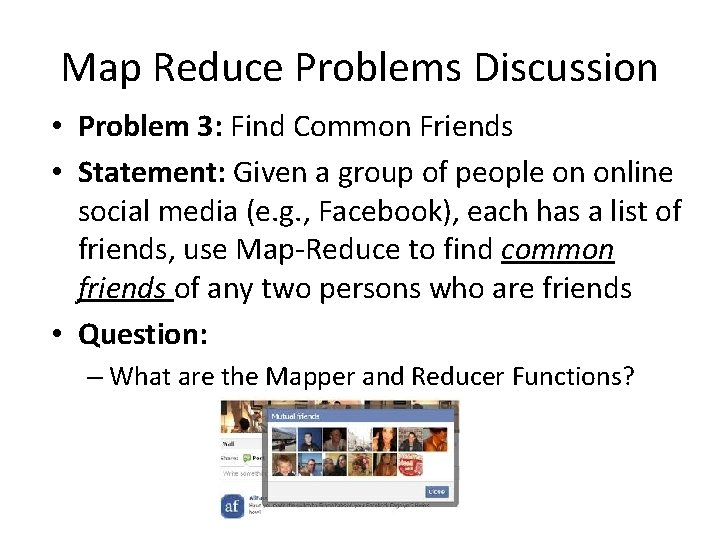Map Reduce Problems Discussion • Problem 3: Find Common Friends • Statement: Given a group of people on online social media (e. g. , Facebook), each has a list of friends, use Map-Reduce to find common friends of any two persons who are friends • Question: – What are the Mapper and Reducer Functions?Map Reduce Problems Discussion • Problem 3: Find Common Friends Input: • Simple example: A B C D A -> B, C, D B-> A, C, D C-> A, B D->A, B Output: (A , B) -> C, D (A, C) -> B (A, D) ->. . …. Map. ReduceMapper and Reducer of Common Friends • Map(key, value){ // key: person_id // value: the list of friends of the person for each friend f_id in value: Emit(<person_id, f_id>, value); } • Reduce(key, list of values){ // key: <friend pair> // list of values: a set of friend lists related with the friend pair for v 1, v 2 in values: common_friends = v 1 intersects v 2; Emit(key, common_friends); }Map Reduce Problems Discussion • Problem 3: Find Common Friends • Mapper and Reducer: – Mapper(friend list of a person) { for each person in the friend list: Emit (<friend pair>, <list of friends>) } – Reducer(output of map) { Emit (<friend pair>, Intersection of two (i. e, the one in friend pair) friend lists)}Map Reduce Problems Discussion • Problem 3: Find Common Friends • Mapper and Reducer: Input: Map: Reduce: A -> B, C, D B-> A, C, D C-> A, B D->A, B (A, B) -> B, C, D (A, C) -> B, C, D (A, D) -> B, C, D (A, B) -> A, C, D (B, C) -> A, C, D (B, D) -> A, C, D (A, C) -> A, B (B, C) -> A, B (A, D) -> A, B (B, D) -> A, B (A, B) -> C, D (A, C) -> B (A, D) -> B (B, C) -> A (B, D) -> A Suggest Fiends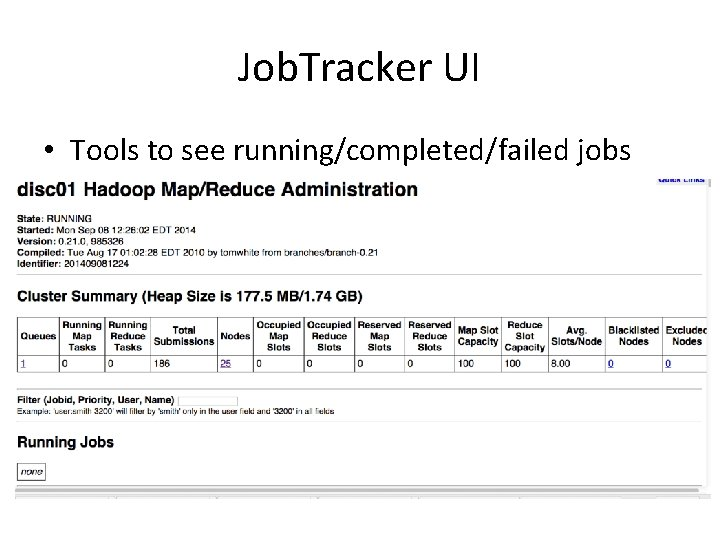Job. Tracker UI • Tools to see running/completed/failed jobs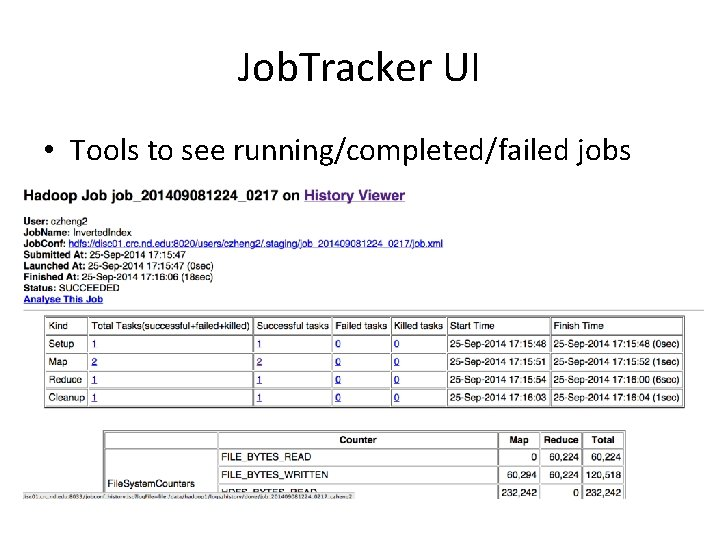Job. Tracker UI • Tools to see running/completed/failed jobs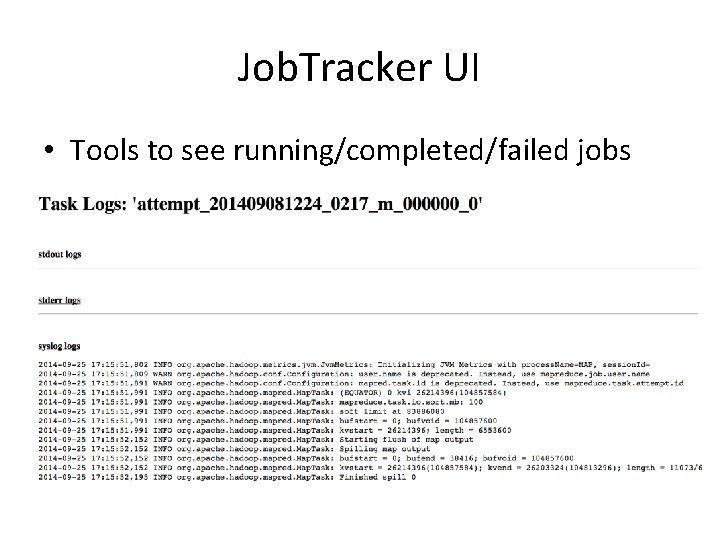Job. Tracker UI • Tools to see running/completed/failed jobs